# Freight train

Twenty-five identical freight wagons are connected behind the locomotive. The weight of the locomotive and all wagons is 1009000 kg. The locomotive weighs 84 tons. How many kilograms does one freight car weigh?

m2 =  37000 kg

### Step-by-step explanation: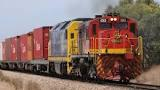Did you find an error or inaccuracy? Feel free to write us. Thank you!Tips to related online calculators
Do you want to convert mass units?

## Related math problems and questions:

• Locomotive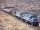The whole loaded train weighs 760 tons. It has twenty wagons, each carrying 20 tons of grain and empty weighs 10 tons. How much weight a locomotive?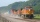The whole loaded train weighs 360 tons. It has twenty wagons, each carrying 12 tons of grain. What is the weight of the locomotive?
• Brick weightThe brick weighs 3 kg and a half-brick. How much does one brick weigh?
• Train tons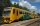The entire loaded train weighs 760 tons. It has a total of 30 wagons, each carrying 12 tons of grain and empty weighs 10 tons. What is the weight of the locomotive?
• One kilogramThe apple weighs 125 grams and half apple. How many apples weigh 1 kilogram?
• Cast brass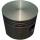Brass cast weight was 3.84 kg, it had turned into piston weight 3.491 kg. How many grams of brass was turned off?
• Tons of coal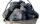Coal hopper has a capacity of 285 liters. How many tons is it? The bulk density of coal is 916 kg/m3.
• Bag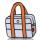Nelly found interesting point. An empty bag weighs 4 kg less than full. And empty bag is 5 times easier than full. How many kg weigh things in this bag?
• Milk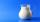Mum bought 16 cartons of milk. One carton of milk weighs 0.925 kg. How many weight all purchase?
• Can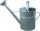Watering can full of water weighs 10 kg. Half-full can weighs 5.5 kg. How much weigh can?
• Weigh in totalI put 3/5 kg of grapes into a box which is 1/4kg in weight. How many kilograms do the grapes and the box weigh in total?
• SandThe maximum weight of the car is 5000 kg. 10 m3 of sand must be transferred. How many times does a car have to go? (density of sand is 1500 kg/m3)
• Bob and Bobek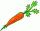Bobek has 2 cabbages and 5 carrots, Bob has 2 cabbages and 9 carrots. When Bobek stepped on the scale weight 5800 g and when Bob weight 8960 g. Actual Bob weight is 8 kg and Bobek 5 kg. How much weighs one carrot?
• Bricks II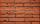3/4 bricks weighs 6 kg and 2/3 bricks. How weighs one whole brick?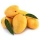A basket contains three types of fruits weighing 87/4 kg in all. If 23/4 kilograms of these are oranges, 48/7 kg are mangoes, and the rest are apples. What is the weight of the apples in the basket?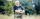Farmers loaded into a truck of fruit and vegetables intended for the store. 10 boxes of 5 kg pears, 8 boxes of 6 kg plums, 7 boxes 9 kg of carrots and 10 bags of 50 kg of potatoes. How many kilograms of fruit and vegetables loaded in total? How many kg coBarrel of water weighs 63 kg. After off 75% water, the weight of the barrel with water is 21 kg. How many kg weigh empty barrel and how many kgs water in it?